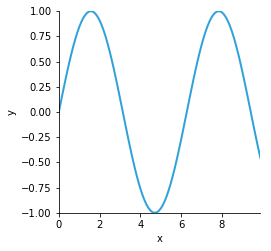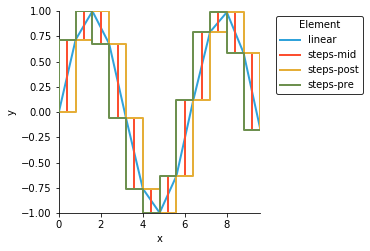# Curve¶

Title
Curve Element
Dependencies
Matplotlib
Backends
Matplotlib
Bokeh
In :
import numpy as np
import holoviews as hv
hv.extension('matplotlib')Curve Elements are used to display quantitative values over a continuous interval or time span. They accept tabular data with one key dimension representing the samples along the x-axis and one value dimension of the height of the curve at for each sample. See the Tabular Datasets user guide for supported data formats, which include arrays, pandas dataframes and dictionaries of arrays.

#### Simple Curve¶

A Curve is a set of values provided for some set of keys from a continuously indexable 1D coordinate system, where the plotted values will be connected up because they are assumed to be samples from a continuous relation.

In :
points = [(0.1*i, np.sin(0.1*i)) for i in range(100)]
hv.Curve(points)

Out:#### Interpolation¶

The Curve also supports the interpolation plot option to determine whether to linearly interpolate the curve values or to draw discrete steps:

In :
overlay = hv.NdOverlay({
interp: hv.Curve(points[::8]).opts(interpolation=interp)
for interp in ['linear', 'steps-mid', 'steps-pre', 'steps-post']})

overlay.opts(legend_position='right')

Out:For full documentation and the available style and plot options, use hv.help(hv.Curve).